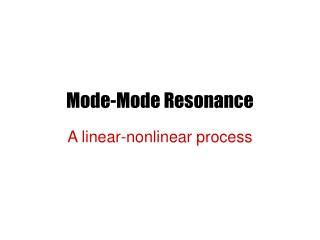DownloadDownload PresentationMode-Mode Resonance

Mode-Mode Resonance

Download PresentationMode-Mode Resonance

- - - - - - - - - - - - - - - - - - - - - - - - - - - E N D - - - - - - - - - - - - - - - - - - - - - - - - - - -
Presentation Transcript

1. Mode-Mode Resonance A linear-nonlinear process

2. Simple Beam Instability • Let us consider • It is well known that the equation supports reactive instability. • What is the cause of instability?

3. One may rewrite the equation It indicates that Langmuir wave is coupled to a beam mode.

4. Consequences depending on nature of coupling • Propagation and evanescence • Convective instability • Absolute instability

5. Mode Evanescence andInstability • Evanescence • Instability

6. Graphical Description Beam mode Complex root

7. Stability and propagation

8. Stability and blocking

9. Convective Instability

10. Convective Instability • The frequency is complex in certain range of k so that the system is unstable. • The roots of the unstable roots are in the same half plane of k. The instability is convective.

11. Absolute Instability

12. Absolute Instability • The frequency is complex in certain range of k so that the system is unstable. • The roots of the unstable roots are in opposite half planes of k. Thus the instability is absolute.

13. Two Other Electron Beam Instabilities • Beam mode coupled with right-hand polarized ion cyclotron wave • Beam mode couple with left-hand polarized ion cyclotron wave

14. Ion cyclotron-beam instability • The dispersion relation is • Coupling of beam-cyclotron mode and the electromagnetic ion cyclotron mode leads to two different instabilities

15. Two electron cyclotron-beam modes Left-hand polarized Right-hand polarized

16. Right-hand polarized beam mode

17. Absolute Instability

18. Left-hand polarized beam mode

19. Convective Instability

20. The two beam instabilities • Have fundamentally different properties. • The right-hand mode is absolutely unstable. • The left-hand mode is convectively unstable

21. Modified Two Stream Instability • The instability is related to shock wave study in the early 1970s. • The instability theory is rather simple and the physics is fairly interesting. • From the viewpoint of mode-coupling process it is obvious.

22. Dispersion Relation • Consider electrostatic waves in a magnetized plasma • Consider and obtain

23. Instability and Growth Rate • Thus we obtain

24. Mode Coupling and Modulation • This is another important process in plasma physics. • It is relevant to parametric excitation of waves.

25. An Oscillator with Modulation • The equation that describes the motion is • The modulation frequency is

26. Physical Parameters • Natural frequency • Pump or modulation frequency • Modulation amplitude • Oscillator with modulation

27. Fourier transform leads to • Two coupled oscillators if where only terms close to the natural frequency are retained. Eventually we obtain the following dispersion equation

28. Two Cases of Interest

29. Dispersion Equation • Eliminating X and Y we obtain the dispersion equation • Two cases of interest

30. Further Discussion Will be given later when we consider parametric instabilities. The details are similar to those discussed earlier.

31. Summary and Conclusions • Mode coupling in general plays important roles. • It can lead to reactive instabilities such as various types of beam instabilities. • The coupled oscillator problem is an introduction of the theory of parametric instability.